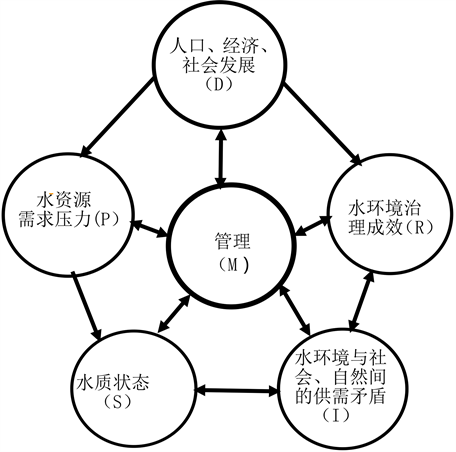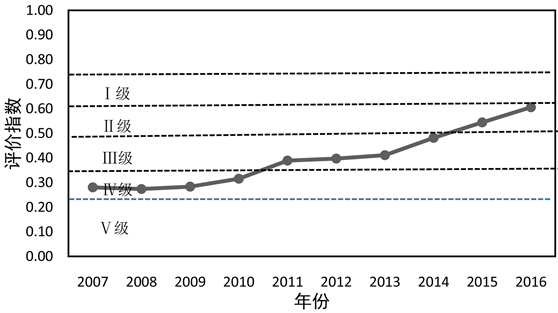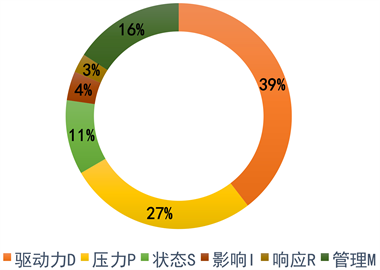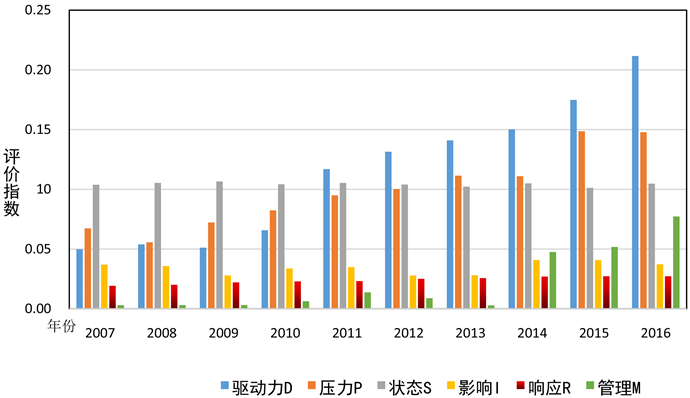# 基于DPSIRM汉江生态经济带水环境承载力评价研究Study on the Evaluation of Water Environment Capacity of Hanjiang River Ecological Economic Belt Based on DPSIRM

• 全文下载: PDF(1413KB)    PP.277-285   DOI: 10.12677/AEP.2019.93039
• 下载量: 118  浏览量: 218   国家科技经费支持

In order to evaluate the water environment carrying capacity of the Hanjiang River eco-economic belt, based on the concept framework of DPSIRM, the evaluation index system of the water envi-ronment carrying capacity was constructed, and the changing trend and influencing factors of the water environment carrying capacity of the Hanjiang River in Hubei province from 2007 to 2016 were analyzed and evaluated systematically. The results show that the overall water environment carrying capacity of the Hubei Hanjiang River eco-economic belt has risen from 2007 to 2016, and the evaluation level has changed from Class IV in 2007 to Grade II in 2016, indicating that the water environment carrying capacity is gradually increasing. The driving force target layer has the highest impact on the water environment carrying capacity, and the evaluation index is the highest, followed by the pressure target layer and management target layer, indicating that the driving force target layer is the most important factor affecting the water carrying capacity of the ecological economic belt of the Hanjiang River in Hubei Province. The six indicator layers that have the greatest impact on the degree of water environment carrying capacity are: per capita GDP, energy consumption per unit of GDP, average water consumption per Mu of farmland irrigation, per capita daily water consumption, per unit of arable land fertilizer application, and environmental pollution management investment in the proportion of GDP. The research results are of theoretical reference value and practical guidance to the improvement of water environment, the rational use of water resources and the construction of ecological environment in Hubei Hanjiang River.

1. 引言

2. 研究区概况与数据来源

2.1. 研究区概况

2.2. 数据来源

3. 研究方法

3.1. 指标体系的构建Figure 1. DPSIRM framework model for water environment carrying capacity

Table 1. Evaluation index system for water environment carrying capacity of Hanjiang ecological economic belt

3.2. 单指标评价指数计算

$Ii=\left\{\begin{array}{cc}0& Xi\text{}XiM\end{array}$ (1)

$Ii=\left\{\begin{array}{cc}1& Xi\text{}XiM\end{array}$ (2)

(式中：Xi为第i个指标的实际值，Xim和XiM分别是为指标i设置的极小值和极大值)。

3.3. 指标权重的确定

${V}_{i}={\sigma }_{i}/{\stackrel{¯}{X}}_{i}$ (3)

${W}_{i}={V}_{i}/{\sum }_{i=1}^{n}{V}_{i}$ (4)

(式中： ${\sigma }_{i}$ 为第i项指标的标准差； ${\stackrel{¯}{X}}_{i}$ 为第i项指标的平均值；Vi为第i项指标的变异系数)。

$F={\sum }_{i=1}^{n}Wi•{I}_{i}$ (5)

(式中：Ii为第i个指标的标准值；Wi为指标i的权重)。

4. 汉江生态经济带水环境承载力评价与分析Figure 2. 2007-2016 water environment carrying capacity evaluation index (F)Figure 3. The weight ratio of each target layerFigure 4. Each target layer evaluation index

5. 结论

NOTES

*通讯作者。

  黄涛珍, 宋胜帮. 淮河流域水环境承载力评价研究[J]. 中国农村水利水电, 2013(4): 45-49.  唐剑武, 郭怀成, 叶文虎. 环境承载力及其在环境规划中的初步应用[J]. 中国环境科学, 1997, 17(1): 6-9.  李姣, 严定容. 湖南省及洞庭湖区重点城市水环境承载力研究[J]. 经济地理, 2013, 33(10): 157-162.  刑有凯, 余红, 肖扬, 等. 基于向量模法的北京市水环境承载力评价[J]. 水资源保护, 2008, 24(4): 1-3+9.  徐志青, 刘雪瑜, 肖书虎, 等. 珠江三角洲地区水环境承载力评价及障碍因素研究[J]. 环境工程技术学报, 2019, 9(1): 44-52.  胡溪, 刘年磊, 蒋洪强, 等. 基于环境质量标准的长江经济带水环境承载力评价[J]. 环境保护, 2018, 46(21): 36-40.  张静, 唐莲, 刘子西, 等. 宁夏水环境承载力变化趋势及影响因素研究[EB/OL]. 宁夏大学学报(自然科学版). http://kns.cnki.net/kcms/detail/64.1006.N.20190403.2124.004.html, 2019-04-21.  蓝希, 刘小琼, 郭炎, 等. “长江经济带”战略背景下武汉城市水环境承载力综合评价[J]. 长江流域资源与环境, 2018, 27(7): 1433-1443.  梁小青, 杜华清, 肖习明. 汉江生态经济带绿色发展思考[J]. 领导科学论坛, 2017(19): 44-45+66.  颜利, 王金坑, 黄浩. 基于PSR框架模型的东溪流域生态系统健康评价[J]. 资源科学, 2008, 30(1): 107-113.  谈迎新, 於忠祥. 基于DSR模型的淮河流域生态安全评价研究[J]. 安徽农业大学学报(社会科学版), 2012, 21(5): 35-39.  陈洋波, 陈俊和, 李长兴, 等. 基于DPSIR模型的深圳市水资源承载能力评价指标体系[J]. 水利学报, 2004, 35(7): 98-103.  郭倩, 汪嘉杨, 张碧. 基于DPSIRM框架的区域水资源承载力综合评价[J]. 自然资源学报, 2017, 32(3): 484-493.  汪嘉杨, 翟庆伟, 郭倩, 等. 太湖流域水环境承载力评价研究[J]. 中国环境科学, 2017, 37(5): 1979-1987.  程大友. 基于变异系数法的财产保险公司绩效评价研究[J]. 改革与战略, 2008, 24(2): 128-130.